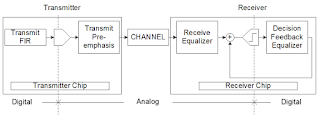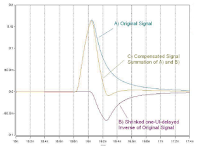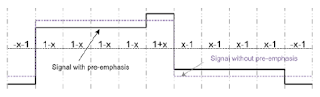## You need to test, we're here to help.

You need to test, we're here to help.

## 27 June 2018

### Introduction to Channel EqualizationFigure 1: Transmit pre-emphasis pre-distorts signals in anticipation of the channel's effects
A number of factors can cause degradation of performance in a high-speed serial data link. Among them are inter-symbol interference (ISI) jitter, attenuation, reflections due to impedance mismatches, and insertion losses, to name a few. But fear not: There are techniques one may use to compensate for these losses known as equalization. We'll review the basics of channel equalization in today's post.Figure 2: Transmit pre-emphasis is better described as "de-emphasis" as bits after the first in each one and zero are decreased in amplitude
One type of equalization is transmit pre-emphasis, in which the transmitted signal is "pre-distorted" by boosting high-frequency transitions relative to low-frequency transitions to compensate for the deleterious effects of the channel (Figure 1). Pre-emphasis comes in two flavors: pre-shoot and de-emphasis. Transmit pre-emphasis is technically not equalization, but it has the same goal of reducing the link's bit-error rate (BER), so it can be thought of as a form of equalization.Figure 3: Pre-emphasis is implemented using an FIR filter to apply delay and inversion to the signal; the result is added back to the original signal to compensate for ISI
After the signal traverses the channel, there are three types of equalization at the receiver: feedforward equalization (FFE), continuous time linear equalization (CTLE), and decision feedback equalization (DFE). Linear filtering at the receiver, accomplished either as a low-pass filter in CTLE or by using an FIR filter, boosts the signal's high-frequency content. DFE is a non-linear filter that also boosts high frequencies but does so after the detector or "slicer."

What does pre-emphasis look like in terms of its effect on the signal as it leaves the transmitter? At left in Figure 2 is a serial-data signal before applying emphasis; at right is the same signal after pre-emphasis, although, in this case, it's actually de-emphasis that's applied. Note that after the first bit in each one and zero, the subsequent bits are de-emphasized with respect to the first bit in terms of amplitude.Figure 4: The FIR filter's three taps are applied pre-, post-, and current-cursor
Pre-emphasis on the transmitted signal is implemented using a multi-tap finite impulse response (FIR) filter, which applies delay and inversion to the signal and then adds it back to the original signal with the proper weight. This is intended to counter the effects of inter-symbol interference due to dispersion by adding the dispersed portion of the signal's energy back to the original signal.

The image in Figure 3 will help illustrate what's going on. The original signal (A at top) is significantly broadened by channel effects. Signal B at bottom is the shrunken, one-UI-delayed inversion of the original signal that's multiplied by a tap coefficient of the FIR filter, and then added back to the original signal. The resulting compensated signal C exhibits diminished pulse broadening and less prominent ISI effects.Figure 5: The FIR filter's pre-cursor tap emphasizes the last bitin a given bit sequence to create pre-shoot
Typically, the FIR filters used to perform transmitter pre-emphasis are three-tap filters. One tap handles pre-cursor ISI, one post-cursor ISI, and one current-cursor ISI, as shown in Figure 4. The effect of the pre-cursor tap (tap C-1 in Figure 4) would be to emphasize the last bit in a given bit sequence as shown in Figure 5. This represents the "pre-shoot" flavor of transmitter pre-emphasis. The post-cursor tap (tap C+1 in Figure 4) emphasizes the first bit of a given bit sequence (Figure 6) and de-emphasizes the rest; hence, it's referred to as "de-emphasis."Figure 6: The FIR filter's post-cursor tap emphasizes the first bitin a given bit sequence to create de-emphasis

The net effect of a three-tap FIR filter is to generate a signal with the proper amounts of de-emphasis and pre-shoot to pre-distort the signal in anticipation of the channel losses it would see. We expect the higher frequencies to be attenuated to a much higher degree by the channel, so we boost those high-frequency components of the signal, which are concentrated on the signal's edges, to pre-emptively cancel out the channel's effects.

We'll continue our discussion of de-emphasis in an upcoming post with some illustrations drawn from a simulation.

Previous posts in this series:

Introduction to Debugging High-Speed Serial Links
A Look at Transmission-Line Losses
How Much Transmission-Line Loss is Too Much?
Inter-Symbol Interference (or Leaky Bits)